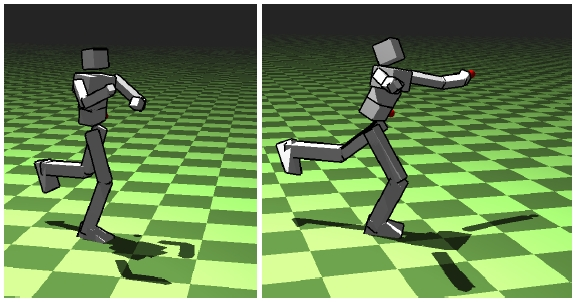EN FR
EN FR

## Section: New Results

### Principal Geodesic Dynamics

This work presents a new integration of a data-driven approach using dimension reduction and a physically-based simulation for real-time character animation (Figure 5 ). We exploit Lie group statistical analysis techniques (Principal Geodesic Analysis, PGA) to approximate the pose manifold of a motion capture sequence by a reduced set of pose geodesics. We integrate this kinematic parametrization into a physically-based animation approach of virtual characters, by using the PGA-reduced parametrization directly as generalized coordinates of a Lagrangian formulation of mechanics. In order to achieve real-time without sacrificing stability, we derive an explicit time integrator by approximating existing variational integrators. Finally, we test our approach in task-space motion control. By formulating both physical simulation and inverse kinematics time stepping schemes as two quadratic programs, we propose a features-based control algorithm that interpolates between the two metrics. This allows for an intuitive trade-off between realistic physical simulation and controllable kinematic manipulation .

Figure 5. Principal Geodesic Dynamics : test of the balance controller